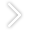# [分享]结构设计中的各种刚度比剖析（上篇）

（1）规范对剪切刚度比的相关要求。

a.高规要求。b.抗规要求。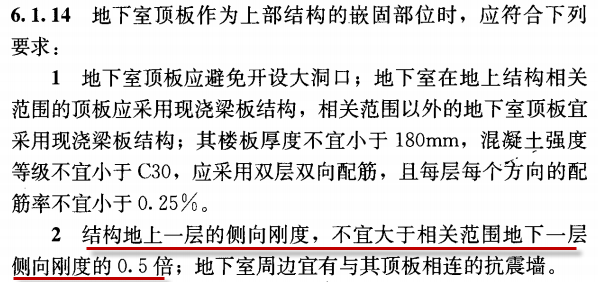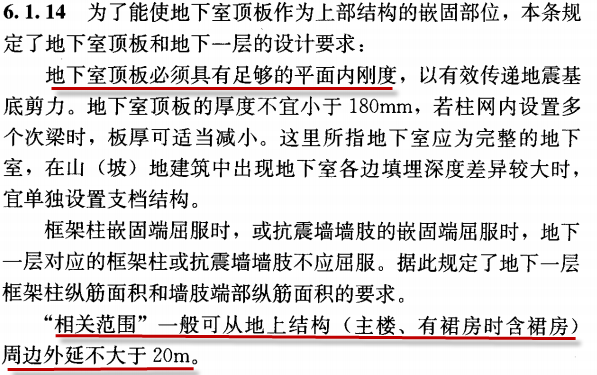c.上海抗规要求。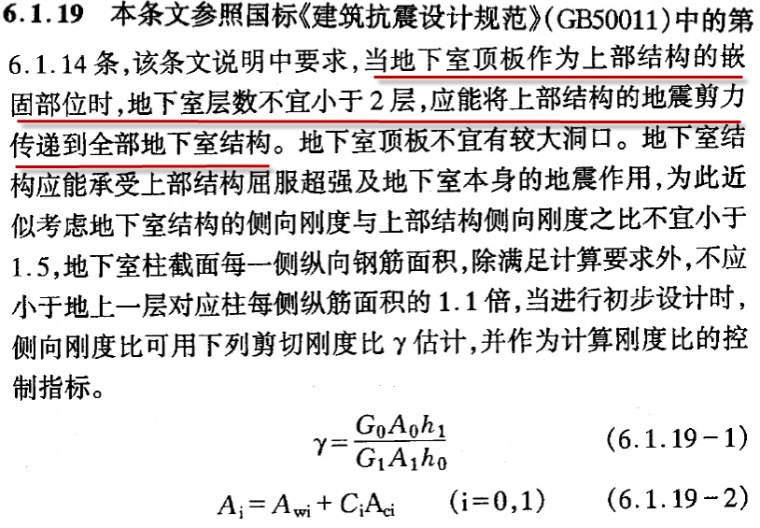d.高规对楼层剪切刚度的计算要求。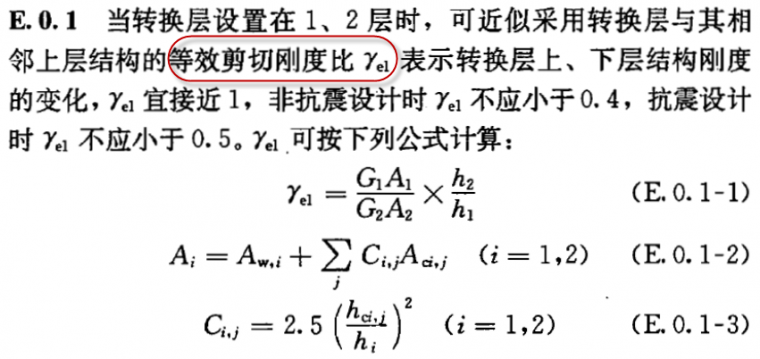（2）对剪切刚度的计算模型选取及结果分析校核。
a. 剪切刚度计算模型的选取。b. 剪切刚度在软件中的计算及校核。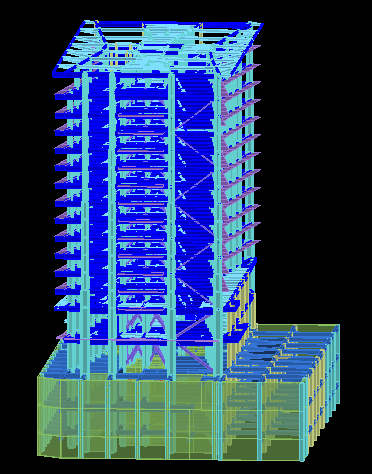X方向剪切刚度为RJX1=4.0548e+7kN/m
Y方向的剪切刚度为RJY1=5.8418+7kN/m。

X方向剪切刚度为RJX1=4.8402e+8kN/m，
Y方向的剪切刚度为RJY1=3.5317+8kN/m。

Ratx=4.0548/（4.8402*10）=0.0838<0.5，
Raty=5.8418/（3.5317*10）=0.1654<0.5。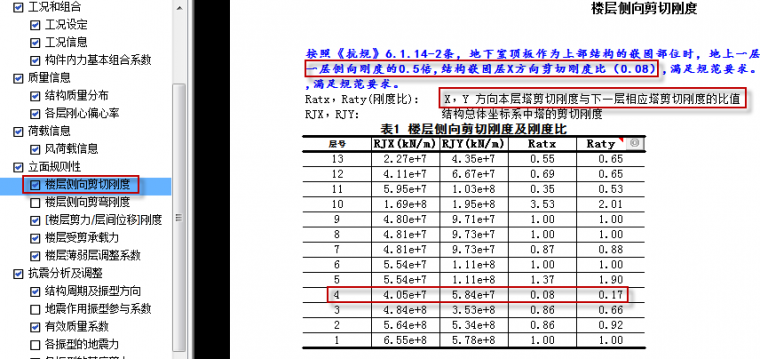X方向剪切刚度RJX1=4.05e+7kN/m，
Y 方向的剪切刚度RJY1=5.84+7kN/m；

X方向剪切刚度RJX1=4.84e+8kN/m，
Y 方向的剪切刚度RJY1=3.53+8kN/m。

RatX=4.05/（4.84*10）=0.08，
RatY=5.84/（3.53*10）=0.17。（1）规范对层剪力与层间位移的刚度比相关要求。

a. 抗规对楼层剪力/层间位移计算的刚度比控制要求。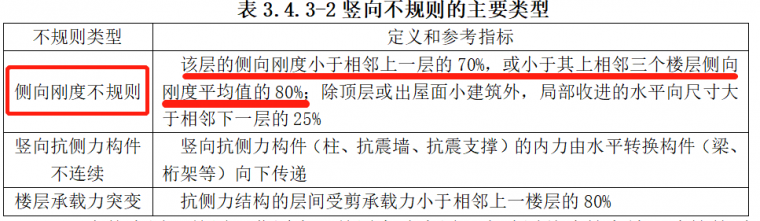b. 高规对楼层剪力与层间位移计算的刚度比控制要求。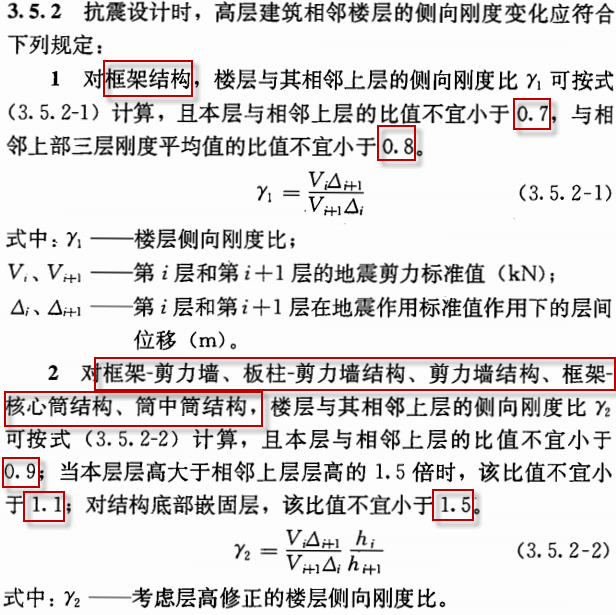（2）对层剪力比层间位移刚度比的计算模型选取及结果分析校核。
a.楼层剪力与层间位移计算刚度比的模型选取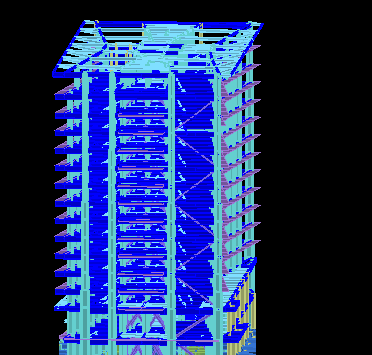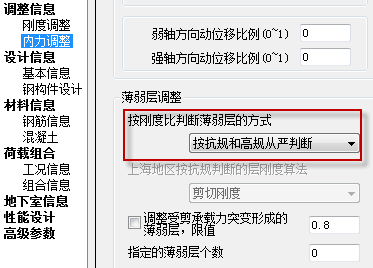b.结构楼层剪力与层间位移计算刚度比的计算和校核b1.手工校核第一层的刚度比。

Ratx1，Raty1分别为按抗规控制的楼层刚度比，即X，Y 方向本层塔侧移刚度与上一层相应塔侧移刚度70%的比值或上三层平均侧移刚度80%的比值中之较小者。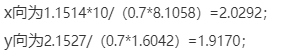Ratx1=min（2.0292,2.1903）=2.0292，
Raty1=min（1.9170,2.144）=1.9170；

Ratx2，Raty2 分别为：X，Y 方向的刚度比，对于非广东地区分框架结构和非框架结构,框架结构刚度比与《抗规》类似,非框架结构为考虑层高修正的刚度比；对于广东地区为考虑层高修正的刚度比(《高规》刚度比)。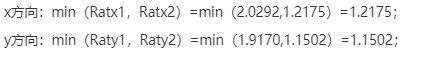b2.手工校核第二层的刚度比：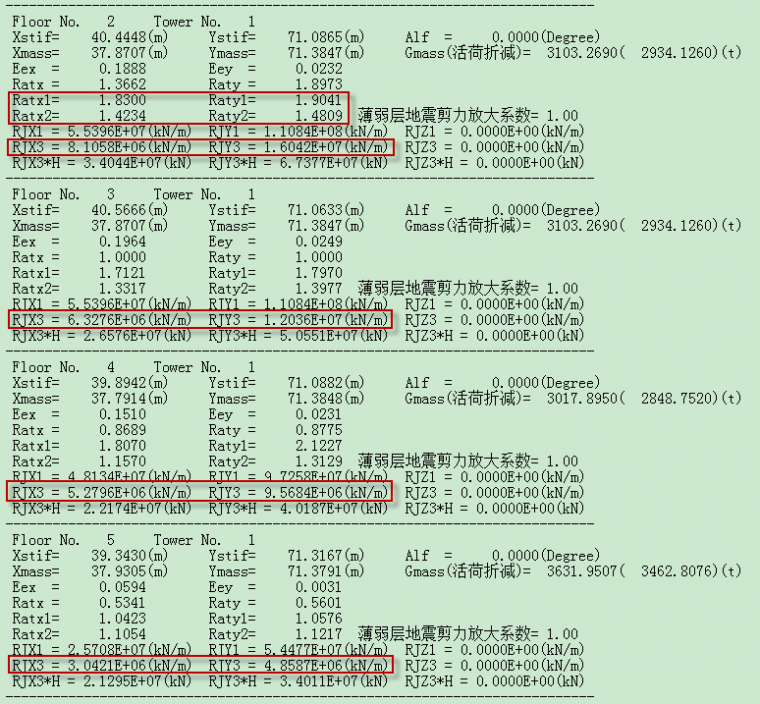Ratx1，Raty1分别为按照抗规控制的楼层刚度比，即X，Y 方向本层塔侧移刚度与上一层相应塔侧移刚度70%的比值或上三层平均侧移刚度80%的比值中之较小者。

x向为8.1058/（0.7*6.3276）=1.8300；
y向为1.6042/（0.7*1.2036）=1.9041；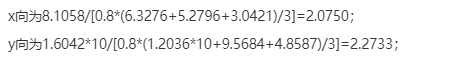Ratx1=min（1.8300,2.0750）= 1.8300，
Raty1=min（1.9041,22733)=1.9041；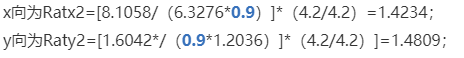b3.手工校核第五层的刚度比：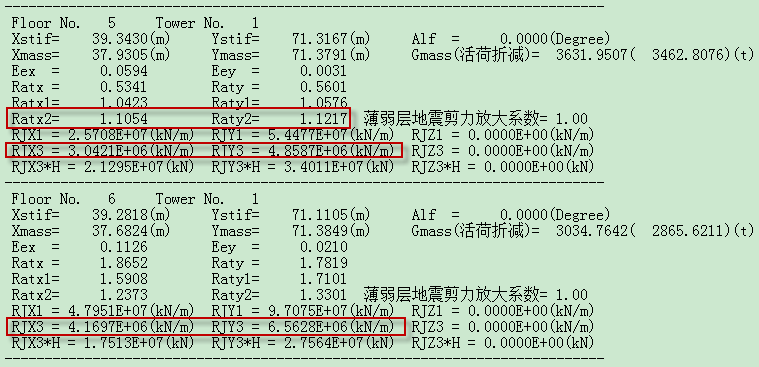RJX3 = 3.0421E+06(kN/m)和RJY3 = 4.8587E+06(kN/m)；

RJX3 = 4.1697E+06(kN/m)和RJY3 = 6.5628E+06(kN/m)；

x向为Ratx2=[3.042/（4.1697*1.1）]*（7/4.2）=1.1054；
y向为Raty2=[4.8587/（1.1*/6.5628）]*（7/4.2）]=1.1217；

（3）新版计算书楼层剪力比层间位移刚度比的结果输出及展示。RJX3 = 1.1514E+07(kN/m)和RJY3 = 2.1527E+07(kN/m)；

RJX3 = 8.1058E+06(kN/m)和RJY3 = 1.6042E+07(kN/m)；

Ratx2=（1.1514*10/8.1058）*（5.4/4.2）=1.826≈1.83；
Raty2=（2.1527/1.6042）*（5.4/4.2）=1.725≈1.73；

Ratx2=（8.1058/6.3276）*（4.2/4.2）=1.281≈1.28；
Raty2=（1.6042/1.2036）*（4.2/4.2）=1.333≈1.33；

Ratx2=（3.042/4.1697）*（7/4.2）=1.2159≈1.22；
Raty2=（4.8587/6.5628）*（7/4.2）=1.234≈1.23；

（4）框架结构楼层剪力比层间位移刚度比的结果输出及校核。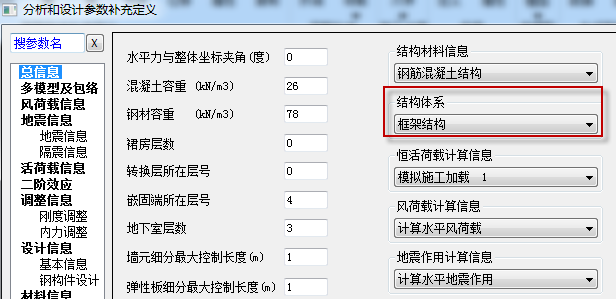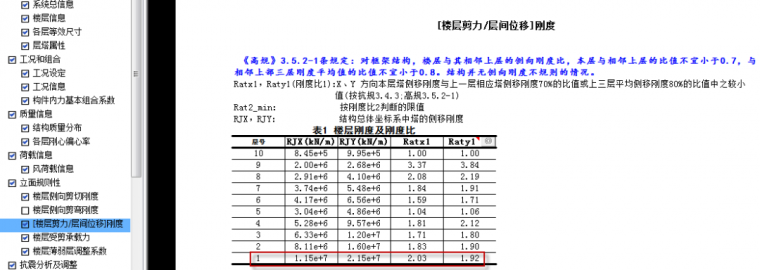发表于2021-01-13   |  只看该作者

2

发表于2021-01-13   |  只看该作者       筑龙币+10

3

### 最新帖子2/20